Question

Updated on:30/05/2023

# A sonar deivce attached to a ship sends. Ultrasomic waves in the sea. These waves are reflected from the bottom of the sea. If the ultrasonic waves take 4 seconds to travel from the ship to the bottom of the sea and back to the ship (in the form of an echol), what is the depth of the sea? (Speed of sound in water =1500m/s).

Text Solution

A
In a hypothetical system , a partical of mass m and charge 3q is moving around a very heavy partical chaRGE q. Assume that Bohr's model is applicable to this system , then velocuity of mass m in the first orbit is 3q22ε0h 3q24ε0h 3q2πε0h 3q24πε0h A mv2r=3q34πε0r2mvr=3q24πε0v (i)
and nh2π=mvr (ii)
Using Bohr's (i) and (ii) and putting n=1
h2π=3q24πε0vv=3q22πε0h The binding energy of nucei X and Y are E1 and E2, respectively. Two atoms of X fuse to give one atom of Y and an energy Q is released. Then, Q=2E1E2 Q=2E22E1 Q<2E1E2 Q>2E22E1 b During fusion, binding energy of daughter nucleus is always greater than the total binding energy of the parent nuclei. The difference of binding energied is released. Hence,
Q=E22E1. A boy is trying to start a fire by focusing sunlight on a piece of paper using an equiconvex lens of focal length 10cm. The diameter of the sun is 1.39×109m and its mean distance from the earth is 1.5×1011m. What is the diameter of the sun's image on the paper ? 9.2×104m 6.5×104m 6.5×105m 12.4×104m A From the relation IO=vu
Here, O=1.39×109,v=0.1m,
u=1.5×1011m
I=0.11.5×1011×1.39×109=9.2×104m The temperature of an open room of volume 30m3 increases from 17C27C due to sunshine. The atmospheric pressure in the room remains 1×105Pa. If niandnf are the number of molecules in the room before and after heating then nf and ni will be 2.5×1025 2.5×1025 1.61×1023 1.38×1023 B Here, V1=30m3,T1=17+273=290K
P1=1×105Pa
V2=30m3,T2=27+273=300K,
P2=1×105Pa.
Let N1,N2 be the no, of moles of a gas at temperature T1andT2 respectively. then
N1=P1V1RT1=(1×105)×3083×290=1.24×103
or N2=P2V2RT2=(1×105)×3083×300=1.20×103 ltbr> Change in the number of moles
N2N1=(1.201.24)×103=0.04×103
Change in the number of molecules
nfni=(N2N1)×(6.023×1023)
=(0.04×103)×(6.023×1023)
=2.5×1025. An ideal monoatomic gas is confined in a horizontal cylinder by a spring loaded piston (as shown in the Fig.) Initially, the gas is at temperature T1,pressureP1andvolumeV1 and the spring is in its relaxed state. The gas is then heated very slowly to temperatureT2,pressureP2andvolumeV2. during this process, the piston moves out by a distance x. ignoring the friction between the piston and the cylinder, the correct statement(s) is (are)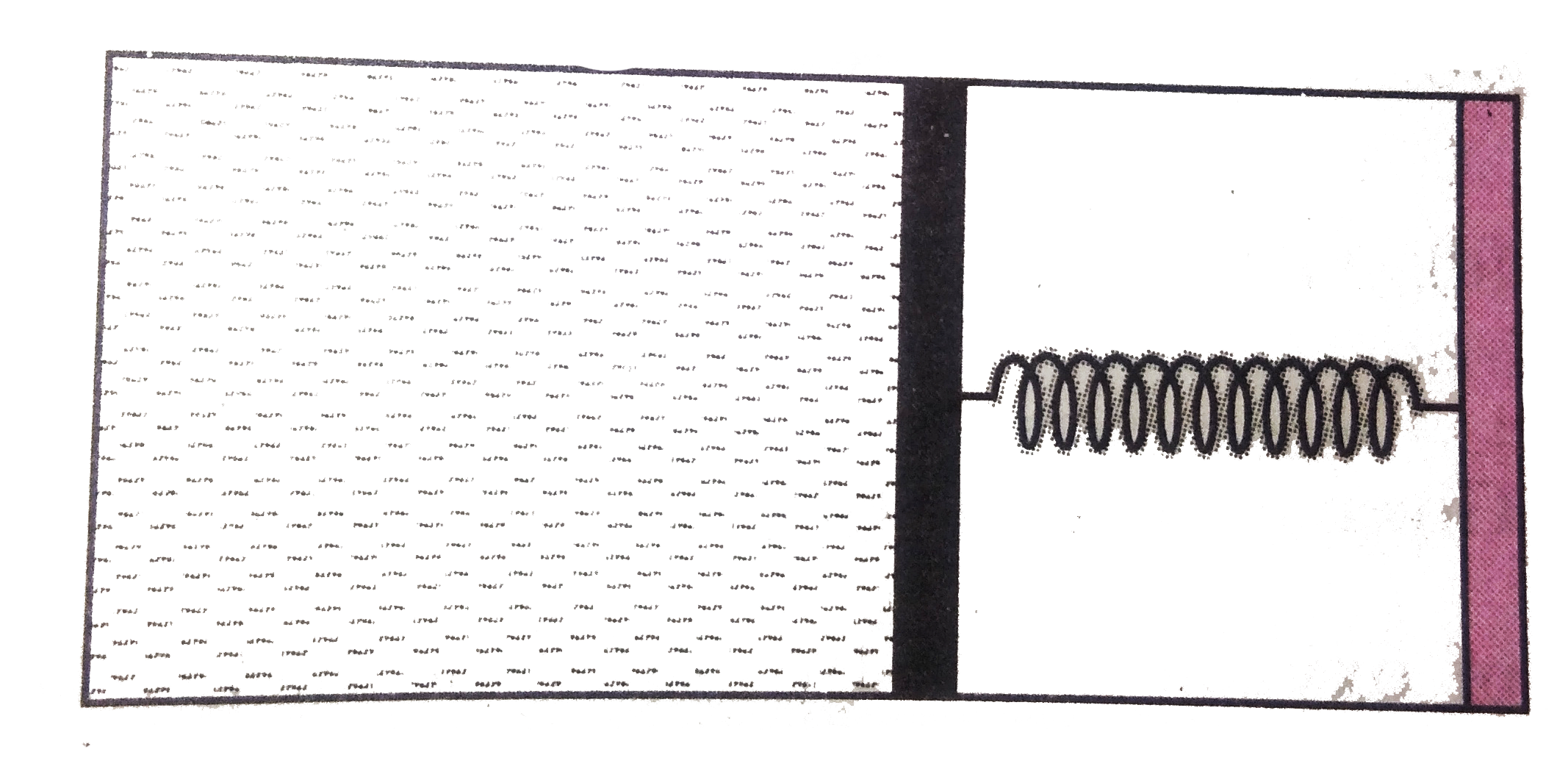If V2=2V1andT2=3T1, then the energy stored in the string is 14P1V1 If V2and2V1 and T2=3T1, then the charge in iternal energy is 3P1V1. If V2=3V1 and T2=4T1, then the work done by the gas is 73P1V1 If V2=3V1 and T2=4T1, then the heat supplied to the gas is 176P1V1. B From gas equation , P1V1T1=P2V2T2=nRorP2=P1V1T2V2T1 ...(i)
(a) when V2=2V1,T2=3T1, then
P2=P1V1×3T12V1×T1=32P1
If A is the area of cross- section of the piston, then
P2A=kxorP2Ax=kx2
Energy in spring , U=12kx2=12P2Ax
=12P2(V2V1)
=12×3P12(2V1V1)=34P1V1
Thus, option (a) is wrong.
(b) When V2=2V1andT2=3T1,P2=32P1 [from (i) ]
Change in internal energy ,
dU=nCvdT=m(3R2)(T2T1)
=n×3R2[3T1T1]=3nRT1=3P1V1
Thus option (b) is true.
(c) when V2=3V1andT2=4T1
P2=P1V1×4T13V1×T1=42P1
Workdone , dW = energy in spring
12kx2=12P2(V2V1)
12×43P1[3V1C1]=43P1V1
Thus option (c) is wrong.
(d) when V2=3V1andT2=4T1,P2=43P1
Heat supplied to the gas, dQ=dU+dW
dQ=n×3R2[T2T1]+12P2(V2V1)
=32nR[4T1T1]+1243P1[3V1V1]
=92nRT1+42P1V1=92P1V1+42P1V1=356P1V1.
Thus, option (d) is wrong. Two rigid boxes containing different ideal gases are placed on a table. Box A contains one mole of nitrogen at T0, while box B contains one mole of He at 7/3T0. The boxes are then put into thermal contact with eachother, and heat flows between them till the gases reach a common final temperature Tf. ignore the heat capacity of boxes. then Tfin terms ofT0 is Tf=73T0 Tf=32T0 Tf=52T0 Tf=37T0 B Here, n1mole,n2=1mole
For nitrogen, Cp1=72R
for helium, Cp2=52R
T1=T0,T2=73T0.Tf=?
when gases are put into thermal contact, heat is exchanged between them till final temperature Tf is reached.
Heat gained by nitrogen = Heat lost by He
n1CP1(TfT1)=n2CP2(T2Tf)
(n1CP1+n2Cp2)Tf=n2Cp2T2n1Cp1T1
(1×7.2R+52R)Tf=1×52R×73T0+1×72RT0
6RTf=(356+72)RT0
Tf=566×6T032T0. If by mistake Ammeter is connected parallel to the resistance then iV curve expected is (Here i= reading of ammeter, V= reading of voltmeter)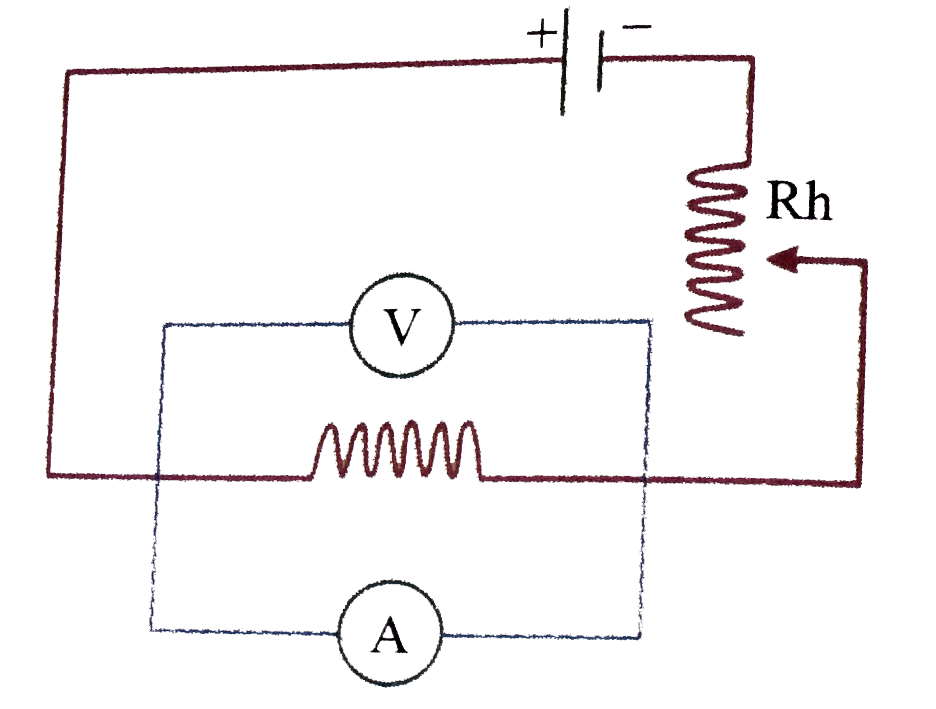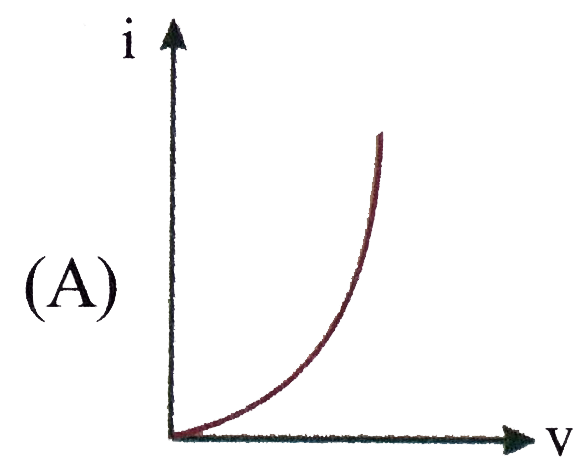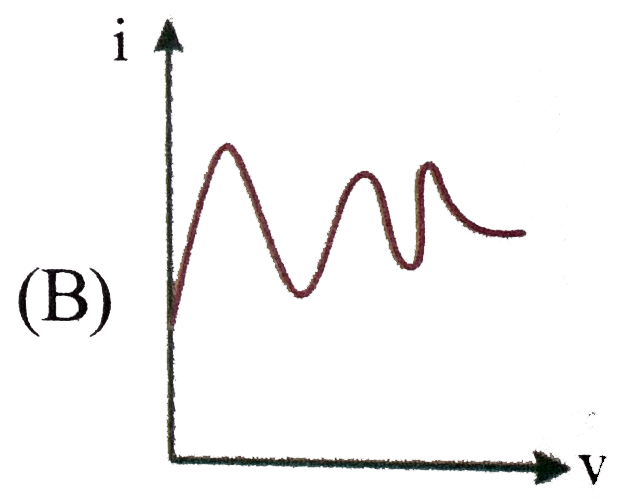C As ammeter has very low resistance most of current will pass through the ammeter so reading of ammeter (i) will be very large. Voltmeter has very high resistance so reading of voltmeter will be very low. Unit of CVρε0 are of
(C= capacitance, V= potential, ρ= specfic resistence and ε0= permittivity of free space) Charge current time frequency B C=ε0Ad and ρ=RAl
Substituting in CVρε0 we get
CVρε0=(ε0Ad)(V)(RAl)ε0current. Two separate monochromatic light beams A and B of the same intensity (energy per unit area per unit time) are falling normally on a unit area of a metallic surface. Their wavelength are λA and λB respectively. Assuming that all the the incident light is used in ejecting the photoelectrons, the ratio of the number of photoelectrons from beam A to that from B is (λAλB) (λBλA) (λAλB)2 (λBλA)2 A The number of photo electron depends on the Number of photons Number of photon =Ihc/λ=λ.Ihcλ
Ratio of no. of photo electrons =λAλB The de Broglie wavelength of an neutron corresponding to root mean square speed at 927 is λ. What will be the de Broglie wavelength of the neutron corresponding to root mean square speed at 27C? λ2 λ 2λ 4λ C K.E. of neutron E=32KT
λd=hp=h2mE=h2m×32kT
λ2=λ(927+273)27+273=2λ A uniform metal rod is used as a bar pendulum. If the room temperature rises by 10C , and the coefficient of linear expansion of the metal of the rod is 2×106 per .C , the period of the pendulum will have percentage increase of 2×103 1×103 2×103 1×103 D If on heating throught 80C , the mass expelled is (1/100)th of mass still remaining, the coefficient of apparent expansion of liquid is 1.25×104/C 12.5×104/C 1.25×105/C None of these A Two identical containers A and B with frictionless pistons contain the same ideal gas at the same temperature and the same volume V . The mass of the gas in A is mAand that in B is mB. The gas in each cylinder is now allowed to expand isothermally to the same final volume 2 V . The changes in the pressure in A and B are found to be ΔPand 1.5 ΔP respectively. Then 4mA=9mB 2mA=3mB 3mA=2mB 9mA=3mB C Following figure shows on adiabatic cylindrical container of volume V0 divided by an adiabatic smooth piston (area of cross-section = A ) in two equal parts. An ideal gas (Cp/Cy=λ) is at pressure P and temperature T in left part and gas at pressure P and temperature T in right part. The piston is slowly displaced and released at a position where it can stay in equilibrium. The final pressure of the two parts will be (Suppose x = displacement of the piston)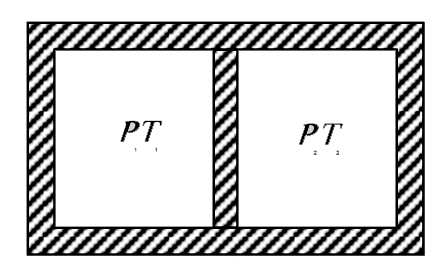P2 P1 P1(V02)λ(V02+Ax)λ P2(V02)λ(V02+Ax)λ C In the following P-V diagram two adiabatics cut two isothermals at temperatures T and T (fig).The value of VaVd will be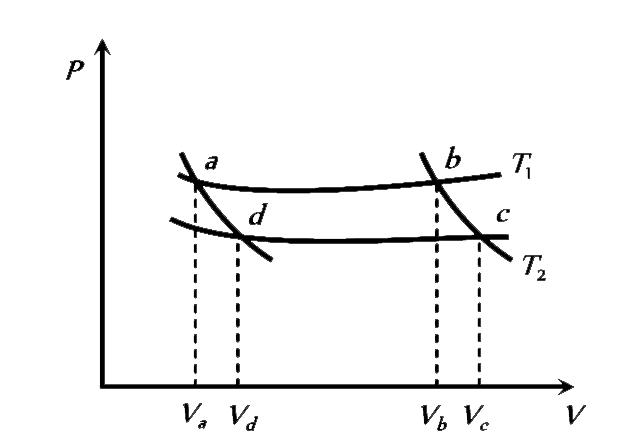VbVc VcVb VdVa VbVe A A light ray from air is incident (as shown in figure) at one end of a glass fiber (refractive index μ=1.5) making an incidence angle of 60 on the lateral surface, so that it undergoes a total internal reflection. How much time would it take to traverse the straight fiber of length 1 km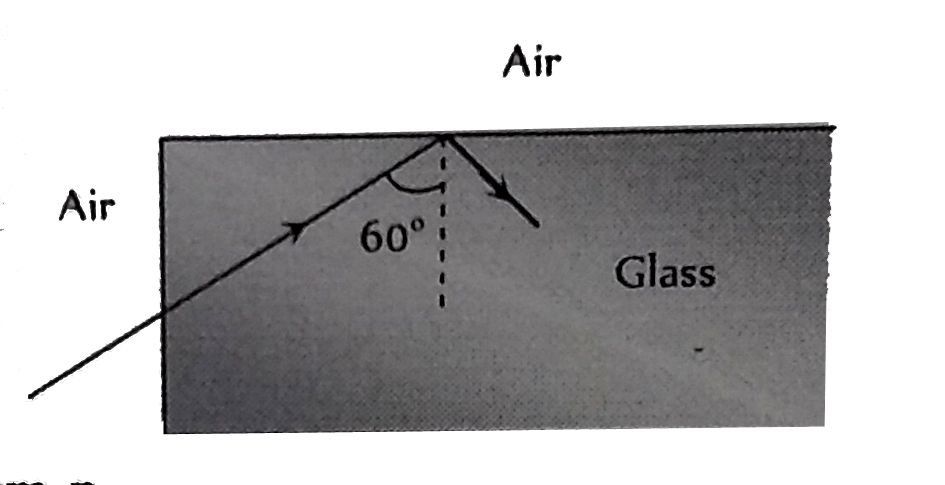3.33μsec 6.67μsec 5.77μsec 3.85μsec D N/A A combination of two thin lenses with focal lengths f1 and f2 respectively forms an image of distant object at distance 60 cm when lenses are in contact. The position of this image shifts by 30 cm towards the combination when two lenses are separated by 10 cm . The corresponding values of f1 and f2 are 30 cm, -60 cm 20 cm, -30 cm 15 cm, -20 cm 12 cm, -15 cm B N/A An optical instrument uses a 25 D objective and 20 D eyepeice with a tube length of 25 cm when eye is least strained The instrument is a telescope with angular magnification 20. The instrument is a microscope with angular magnification 20. The instrument is a telescope with angular magnification 24. The instrument is a microscope with angular magnification 24. B N/A The ratio of d-Brogile wavelength off mlecules of hydrogen and helium gas moving at arms speed in two gas jars kept sparately at temeperature 37 C and 127 C respectively is : 23 23 32 83 D At t=O, light of intensity 1012 photons/sm2 of energy 6eV per photon starts falling on a plate with work founction 2.5eV If area of the plate is 2×104m2 and for every 105 photons one photoelectron is emitted, charge on the plate at t=25 s is 8×1015C 4×1014C 12×1010C 16×1012C A Radius of an electron moving in a circle in constant magnetic field is two times that of an α particle in the same field. Then de-Broglie wavelength of electrons is x-times of the α-particle Here x is 1 A telescope has an objective lens of 10cm diameter and is situated at a distance of one kilometre from two objects. The minimum distance between these two objects, which can be resolved by the telescope, when the mean wavelength of light is 5000Ã, of the order of mm is : 5 Resolving limit of telescope is
θxD=λdx=λDd
Given, λ=5000Ã=5000×101m
D=1km=1000m
d=10cm=0.1m
Hence, x=5000×1010×10000.1
=5×103m=5mm A spring is placed between the jaws of screw gauge such that the spring is not all compressed. The mai scale reads 2 division and circular scale reads 28 divisions. Now we turn the circular scale by 18 such that the spring is compessed. The circular scale has 200 divisions and the least count of the main scale is 1mm. the force exerted by the spring on the jaws in unit mN. (the spring constnat is 100N/m) 5 Assuming human pupil to have a radius of 0.25 cm and a comfortable viewing distance of 25 cm, the minimum separation between two objects than human eye can resolve at 500nm wavelength is Xmm , so value of x is: 30
sinθ=0.2525=1100
Resolving power =1.22λ2μsinθ=30μm In a cyclic process shown in the figure an ideal gas is adiabatically taken from B and A, the work done on the gas during the process BA is 30J, when the gas is taken from AB the heat absorbed by the gas is 20J. The change in internal energy of the gas in the process AB is given as X, so value of X is - :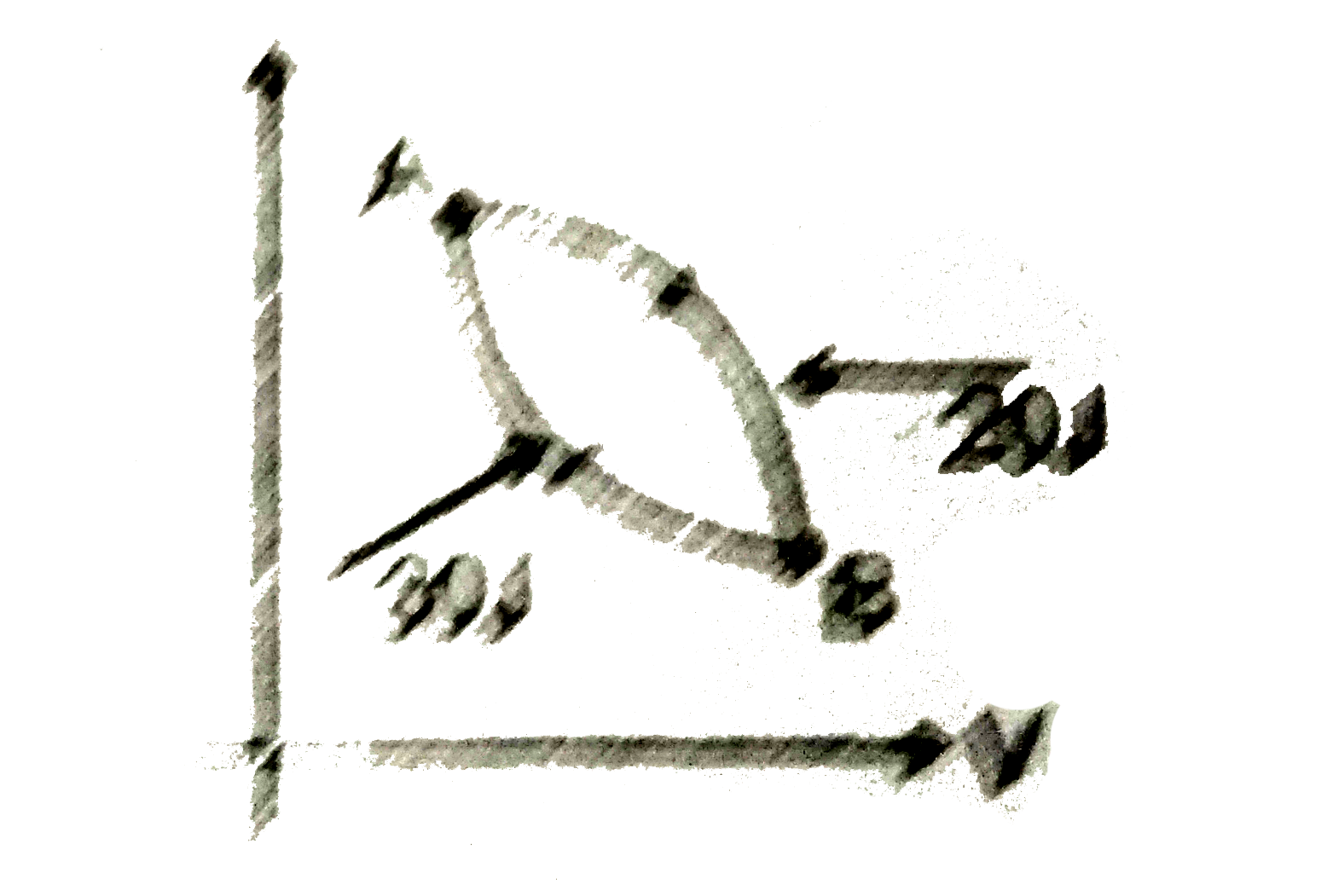30 N//A
Solution
The time taken by the ultrasonic sound waves to travel from the ship to the sea-bed, and back to the ship is 4 seconds. So, the time taken by the ultrasonic sound to travel from the ship to sea-bed will be half of this time, which is 42=2 second. This means that the sound takes 2 seconds to travel from the ship to the bottom of the sea.
Now, Speed =DistanceTime
So, 1500=Distance2
And, Distance =1500×2m=3000m
Thus, the depth of this sea below the ship is 3000 metres.